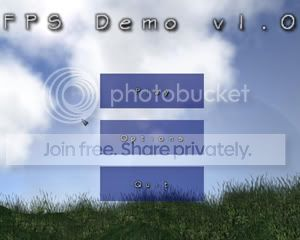-=+=- -=+=- -=+=- -=+=- -=+=- -=+=- -=+=- -=+=- -=+=- -=+=- -=+=- -=+=- -=+=- -=+=- -=+=- -=+=- -=+=- -=+=- -=+=- -=+=- -=+=- -=+=- -=+=- -=+=- -=+=- -=+=- -=+=- -=+=- -=+=- -=+=- (c) WidthPadding Industries 1987 0|291|0 -=+=- -=+=- -=+=- -=+=- -=+=- -=+=- -=+=- -=+=- -=+=- -=+=- -=+=- -=+=- -=+=- -=+=- -=+=- -=+=- -=+=- -=+=- -=+=- -=+=- -=+=- -=+=- -=+=- -=+=- -=+=- -=+=- -=+=- -=+=- -=+=- -=+=-
SoCoder -> Showcase Home -> Graphic Demos

ToasterCreated : 09 October 2007
Edited : 11 October 2007
System : Windows
Language : Blitz

### {WIP} FPS Alpha Demo

Alpha demo for a coming FPS game.

https://nanotech.pcriot.com
https://www.mediafire.com/?3cmjj6igjcs
ScreenshotsI've been working on a FPS right now we got no name but were working on that! Razz

Story First Draft:

Completed Features:
-Own map format
-Particles
-BumpMapping
-Dynamic 3D Clouds
-Dynamic Grass
-Supports older computers

-Better Story
-Maps
-Models(People,aliens,Guns,MotherShip,ect)
-Console (Maybe some secret cheats as well)
-Map Editor
-User Ideas

The public alpha is here!

This version has Realtime Shadows and all sorts of neat features:
www.mediafire.com/?3cmjj6igjcs

Also the field of view is fixed along with the speed that the player moves.

Minimum system requirements:
CPU: 700 MHZ or above
Memory: 256 MB or higher
GFX card with at least 8MB memory
20 MB of free harddrive space

To view at full quality:
CPU: 2.6GHZ or above
Memory:512 MB or above
GFX card with at least 128 MB of memory or more
20 MB of free harddrive space

Mouse to move the view...
Keys:
W=forwards
S=backwards
D=strafe right
A=strafe left
Space=jump
Shift=Move Faster
F1=view/hide framerate and stats

Note in the next release I'll make it so you can map your own keys!

A Public beta will be release around Christmas time! Very Happy The website has more info please check it out and signup for the forums!

nanotech.pcriot.com

-Toaster